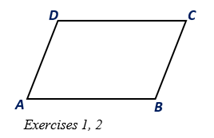Chapter 4.1, Problem 2E### Elementary Geometry for College St...

6th Edition
Daniel C. Alexander + 1 other
ISBN: 9781285195698

#### Solutions

Chapter
Section### Elementary Geometry for College St...

6th Edition
Daniel C. Alexander + 1 other
ISBN: 9781285195698
Textbook Problem
1 views

# ABCD is a parallelogram.a) Using a ruler, compare the lengths of sides A D - and B C - .b) Using a protractor, compare the measures of∠B and ∠D.To determine

a.

To compare:

The lengths of sides AD- and BC- in the parallelogram ABCD using a ruler.

Explanation

Given:

ABCD is a parallelogram.

Corollary:

The opposite sides of a parallelogram are congruent.

From the given parallelogram ABCD, the sides AD- and BC- are a pair of opposite sides

To determine

b.

To compare:

The measures of ∠B and ∠D in a parallelogram by a protractor.

### Still sussing out bartleby?

Check out a sample textbook solution.

See a sample solution

#### The Solution to Your Study Problems

Bartleby provides explanations to thousands of textbook problems written by our experts, many with advanced degrees!

Get Started

#### In Exercises 2932, find the value(s) of x that satisfy the expression. 29. 2x2 + 3x 2 0

Applied Calculus for the Managerial, Life, and Social Sciences: A Brief Approach

#### In Problems 1-4, find the derivative and simplify. 2.

Mathematical Applications for the Management, Life, and Social Sciences

#### In Exercises 516, evaluate the given quantity. log100,000

Finite Mathematics and Applied Calculus (MindTap Course List)

#### Differentiate. F(y)=(1y23y4)(y+5y3)

Single Variable Calculus: Early Transcendentals, Volume I

#### Finding a Limit In Exercises 21-24, find the limit limxtanhx

Calculus: Early Transcendental Functions (MindTap Course List)